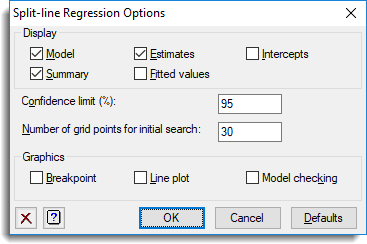1. Home
2. Split-line Regression Options

# Split-line Regression Options

Use this to set options for a split-line regression.## Display

Specifies the output to be displayed.

 Model Displays the model. Summary Summary of the analysis. Estimates Displays estimates of the parameters and confidence interval for the x location of the intersection (or breakpoint) of the lines. Fitted values Displays the fitted values. Intercepts Displays the x-value at which the model intercepts the y-axis, and the y-value at which it intercepts the x-axis.

## Confidence limit (%)

Specifies the confidence limit for the x location of the intersection (or breakpoint) of the lines. This value should be supplied as a percentage.

Specifies the number of grid points used in the initial search for the best point.

## Graphics

Specifies the plots to be displayed.

 Breakpoint Partial likelihood plot displaying the approximate F ratio for the model for a range of positions of the breakpoint between the lines. Lines plot Lines plot of the fitted lines. Model-checking Composite plot of a histogram, Normal plot, half-Normal plot and residuals against fitted values.